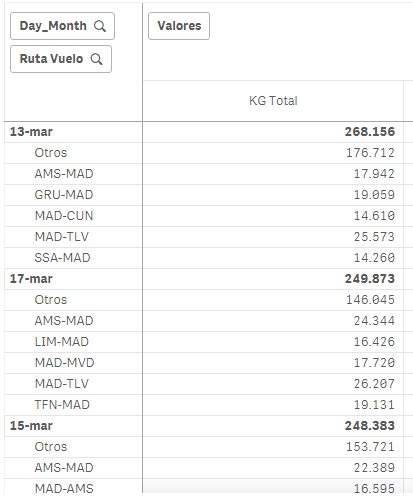Announcements
Applications are open for the 2024 Qlik Luminary Program. Apply by December 15 here.
cancel
Showing results for
Did you mean:Contributor III

## Sorting 2nd dimension Issue

Hi,

I'm having issues to sort this pivot table (also a stacked bar chart).

Sorting shoud be: dates with higher KG_TOTAL and then routes operating on each date also sorted from high to low

Day_Month sort order is working well, I use this expression:

rank(sum(\$(v.Aux_CYTD)
KG_TOTAL))

But I can't get route sort order to work as you can see:Which expression shoud I use to sort by second dimension (Route)?

Jose M.

Labels (1)
• ### Sort by expression

1 Solution

Accepted SolutionsMVP

Hi, field values are sorted globally, so if 'AMS-MAD' it's the second across all dimensions it will show the second in all dimensions, because that's the sort index for that field value.

One workaround to this can be using a calculated dimension that gives a different value to each dimension combination. PFA.

2 RepliesMVP

Hi, field values are sorted globally, so if 'AMS-MAD' it's the second across all dimensions it will show the second in all dimensions, because that's the sort index for that field value.

One workaround to this can be using a calculated dimension that gives a different value to each dimension combination. PFA.Contributor III
Author

Hi Ruben,

It works perfect with your solution

ps.

I haven't seen this expression on Dim2 before:

=Aggr(Dual(Dim2, RowNo(TOTAL)), Dim1, Dim2)